Related Articles

# Pointer to an Array | Array Pointer

• Difficulty Level : Medium
• Last Updated : 21 Sep, 2021

Prerequisite: Pointers Introduction

Pointer to Array

Consider the following program:

## C++

 `#include ``using` `namespace` `std;` `int` `main()``{``int` `arr = { 1, 2, 3, 4, 5 };``int` `*ptr = arr;` `cout <<``"\n"``<< ptr;``return` `0;``}` `// thus code is contributed by shivanisinghss2110`

## C

 `#include` `int` `main()``{``  ``int` `arr = { 1, 2, 3, 4, 5 };``  ``int` `*ptr = arr;` `  ``printf``(``"%p\n"``, ptr);``  ``return` `0;``}`

In this program, we have a pointer ptr that points to the 0th element of the array. Similarly, we can also declare a pointer that can point to whole array instead of only one element of the array. This pointer is useful when talking about multidimensional arrays.
Syntax:

`data_type (*var_name)[size_of_array];`

Example:

`int (*ptr);`

Here ptr is pointer that can point to an array of 10 integers. Since subscript have higher precedence than indirection, it is necessary to enclose the indirection operator and pointer name inside parentheses. Here the type of ptr is ‘pointer to an array of 10 integers’.
Note : The pointer that points to the 0th element of array and the pointer that points to the whole array are totally different. The following program shows this:

## C++

 `// C++ program to understand difference between``// pointer to an integer and pointer to an``// array of integers.``#include ``using` `namespace` `std;``int` `main()``{``    ``// Pointer to an integer``    ``int` `*p;``    ` `    ``// Pointer to an array of 5 integers``    ``int` `(*ptr);``    ``int` `arr;``    ` `    ``// Points to 0th element of the arr.``    ``p = arr;``    ` `    ``// Points to the whole array arr.``    ``ptr = &arr;``    ` `    ``cout << ``"p ="` `<< p <<``", ptr = "``<< ptr<< endl;``    ``p++;``    ``ptr++;``    ``cout << ``"p ="` `<< p <<``", ptr = "``<< ptr<< endl;``    ` `    ``return` `0;``}` `// This code is contributed by SHUBHAMSINGH10`

## C

 `// C program to understand difference between``// pointer to an integer and pointer to an``// array of integers.``#include` `int` `main()``{``    ``// Pointer to an integer``    ``int` `*p;``    ` `    ``// Pointer to an array of 5 integers``    ``int` `(*ptr);``    ``int` `arr;``    ` `    ``// Points to 0th element of the arr.``    ``p = arr;``    ` `    ``// Points to the whole array arr.``    ``ptr = &arr;``    ` `    ``printf``(``"p = %p, ptr = %p\n"``, p, ptr);``    ` `    ``p++;``    ``ptr++;``    ` `    ``printf``(``"p = %p, ptr = %p\n"``, p, ptr);``    ` `    ``return` `0;``}`

Output:

```p = 0x7fff4f32fd50, ptr = 0x7fff4f32fd50
p = 0x7fff4f32fd54, ptr = 0x7fff4f32fd64```

p: is pointer to 0th element of the array arr, while ptr is a pointer that points to the whole array arr

• The base type of p is int while base type of ptr is ‘an array of 5 integers’.
• We know that the pointer arithmetic is performed relative to the base size, so if we write ptr++, then the pointer ptr will be shifted forward by 20 bytes.

The following figure shows the pointer p and ptr. Darker arrow denotes pointer to an array.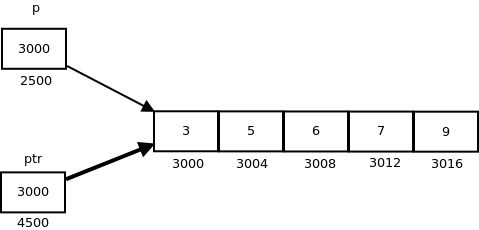On dereferencing a pointer expression we get a value pointed to by that pointer expression. Pointer to an array points to an array, so on dereferencing it, we should get the array, and the name of array denotes the base address. So whenever a pointer to an array is dereferenced, we get the base address of the array to which it points.

## C++

 `// C++ program to illustrate sizes of``// pointer of array``#include ``using` `namespace` `std;` `int` `main()``{``    ``int` `arr[] = { 3, 5, 6, 7, 9 };``    ``int` `*p = arr;``    ``int` `(*ptr) = &arr;``    ` `    ``cout << ``"p = "``<< p <<``", ptr = "` `<< ptr << endl;``    ``cout << ``"*p = "``<< *p <<``", *ptr = "` `<< *ptr << endl;``    ` `    ``cout << ``"sizeof(p) = "``<< ``sizeof``(p) <<``            ``", sizeof(*p) = "` `<< ``sizeof``(*p) << endl;``    ``cout << ``"sizeof(ptr) = "``<< ``sizeof``(ptr) <<``        ``", sizeof(*ptr) = "` `<< ``sizeof``(*ptr) << endl;``    ``return` `0;``}` `// This code is contributed by shubhamsingh10`

## C

 `// C program to illustrate sizes of``// pointer of array``#include` `int` `main()``{``    ``int` `arr[] = { 3, 5, 6, 7, 9 };``    ``int` `*p = arr;``    ``int` `(*ptr) = &arr;``    ` `    ``printf``(``"p = %p, ptr = %p\n"``, p, ptr);``    ``printf``(``"*p = %d, *ptr = %p\n"``, *p, *ptr);``    ` `    ``printf``(``"sizeof(p) = %lu, sizeof(*p) = %lu\n"``,``                          ``sizeof``(p), ``sizeof``(*p));``    ``printf``(``"sizeof(ptr) = %lu, sizeof(*ptr) = %lu\n"``,``                        ``sizeof``(ptr), ``sizeof``(*ptr));``    ``return` `0;``}`

Output:

```p = 0x7ffde1ee5010, ptr = 0x7ffde1ee5010
*p = 3, *ptr = 0x7ffde1ee5010
sizeof(p) = 8, sizeof(*p) = 4
sizeof(ptr) = 8, sizeof(*ptr) = 20```

Pointer to Multidimensional Arrays:

• Pointers and two dimensional Arrays: In a two dimensional array, we can access each element by using two subscripts, where first subscript represents the row number and second subscript represents the column number. The elements of 2-D array can be accessed with the help of pointer notation also. Suppose arr is a 2-D array, we can access any element arr[i][j] of the array using the pointer expression *(*(arr + i) + j). Now we’ll see how this expression can be derived.
Let us take a two dimensional array arr

`int arr = { {1, 2, 3, 4}, {5, 6, 7, 8}, {9, 10, 11, 12} };`Since memory in a computer is organized linearly it is not possible to store the 2-D array in rows and columns. The concept of rows and columns is only theoretical, actually, a 2-D array is stored in row-major order i.e rows are placed next to each other. The following figure shows how the above 2-D array will be stored in memory.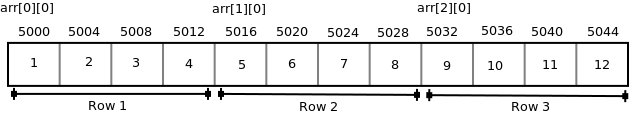Each row can be considered as a 1-D array, so a two-dimensional array can be considered as a collection of one-dimensional arrays that are placed one after another. In other words, we can say that 2-D dimensional arrays that are placed one after another. So here arr is an array of 3 elements where each element is a 1-D array of 4 integers.
We know that the name of an array is a constant pointer that points to 0th 1-D array and contains address 5000. Since arr is a ‘pointer to an array of 4 integers’, according to pointer arithmetic the expression arr + 1 will represent the address 5016 and expression arr + 2 will represent address 5032.
So we can say that arr points to the 0th 1-D array, arr + 1 points to the 1st 1-D array and arr + 2 points to the 2nd 1-D array.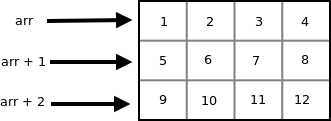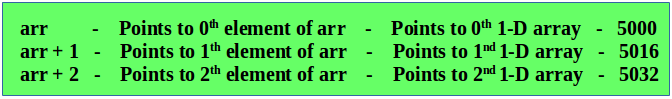In general we can write:

```arr + i  Points to ith element of arr ->
Points to ith 1-D array```

• Since arr + i points to ith element of arr, on dereferencing it will get ith element of arr which is of course a 1-D array. Thus the expression *(arr + i) gives us the base address of ith 1-D array.
• We know, the pointer expression *(arr + i) is equivalent to the subscript expression arr[i]. So *(arr + i) which is same as arr[i] gives us the base address of ith 1-D array.
• To access an individual element of our 2-D array, we should be able to access any jth element of ith 1-D array.
• Since the base type of *(arr + i) is int and it contains the address of 0th element of ith 1-D array, we can get the addresses of subsequent elements in the ith 1-D array by adding integer values to *(arr + i).
• For example *(arr + i) + 1 will represent the address of 1st element of 1stelement of ith 1-D array and *(arr+i)+2 will represent the address of 2nd element of ith 1-D array.
• Similarly *(arr + i) + j will represent the address of jth element of ith 1-D array. On dereferencing this expression we can get the jth element of the ith 1-D array.
• Pointers and Three Dimensional Arrays
In a three dimensional array we can access each element by using three subscripts. Let us take a 3-D array-

`int arr = { {{5, 10}, {6, 11}, {7, 12}}, {{20, 30}, {21, 31}, {22, 32}} };`

We can consider a three dimensional array to be an array of 2-D array i.e each element of a 3-D array is considered to be a 2-D array. The 3-D array arr can be considered as an array consisting of two elements where each element is a 2-D array. The name of the array arr is a pointer to the 0th 2-D array.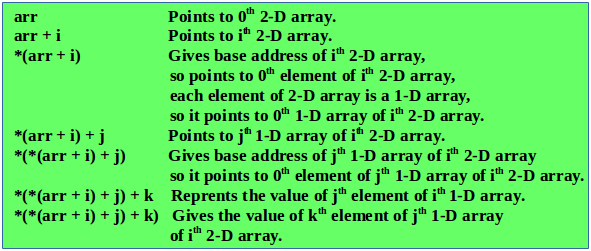Thus the pointer expression *(*(*(arr + i ) + j ) + k) is equivalent to the subscript expression arr[i][j][k].
We know the expression *(arr + i) is equivalent to arr[i] and the expression *(*(arr + i) + j) is equivalent arr[i][j]. So we can say that arr[i] represents the base address of ith 2-D array and arr[i][j] represents the base address of the jth 1-D array.

## C++

 `// C++ program to print the elements of 3-D``// array using pointer notation``#include ``using` `namespace` `std;``int` `main()``{``  ``int` `arr = {``                       ``{``                         ``{5, 10},``                         ``{6, 11},``                         ``{7, 12},``                       ``},``                       ``{``                         ``{20, 30},``                         ``{21, 31},``                         ``{22, 32},``                       ``}``                     ``};``  ``int` `i, j, k;``  ``for` `(i = 0; i < 2; i++)``  ``{``    ``for` `(j = 0; j < 3; j++)``    ``{``       ``for` `(k = 0; k < 2; k++)``          ``cout << *(*(*(arr + i) + j) +k) << ``"\t"``;``       ``cout <<``"\n"``;``    ``}``  ``}` `  ``return` `0;``}` `// this code is contributed by shivanisinghss2110`

## C

 `// C program to print the elements of 3-D``// array using pointer notation``#include``int` `main()``{``  ``int` `arr = {``                       ``{``                         ``{5, 10},``                         ``{6, 11},``                         ``{7, 12},``                       ``},``                       ``{``                         ``{20, 30},``                         ``{21, 31},``                         ``{22, 32},``                       ``}``                     ``};``  ``int` `i, j, k;``  ``for` `(i = 0; i < 2; i++)``  ``{``    ``for` `(j = 0; j < 3; j++)``    ``{``       ``for` `(k = 0; k < 2; k++)``         ``printf``(``"%d\t"``, *(*(*(arr + i) + j) +k));``       ``printf``(``"\n"``);``    ``}``  ``}` `  ``return` `0;``}`

Output:

```5    10
6    11
7    12
20    30
21    31
22    32```

The following figure shows how the 3-D array used in the above program is stored in memory.Subscripting Pointer to an Array

Suppose arr is a 2-D array with 3 rows and 4 columns and ptr is a pointer to an array of 4 integers, and ptr contains the base address of array arr

```int arr = {{10, 11, 12, 13}, {20, 21, 22, 23}, {30, 31, 32, 33}};
int (*ptr);
ptr = arr;```Since ptr is a pointer to an array of 4 integers, ptr + i will point to ith row. On dereferencing ptr + i, we get base address of ith row. To access the address of jth element of ith row we can add j to the pointer expression *(ptr + i). So the pointer expression *(ptr + i) + j gives the address of jth element of ith row and the pointer expression *(*(ptr + i)+j) gives the value of the jth element of ith row.
We know that the pointer expression *(*(ptr + i) + j) is equivalent to subscript expression ptr[i][j]. So if we have a pointer variable containing the base address of 2-D array, then we can access the elements of array by double subscripting that pointer variable.

## C++

 `// C++ program to print elements of a 2-D array``// by scripting a pointer to an array``#include ``using` `namespace` `std;` `int` `main()``{``  ``int` `arr = {``                    ``{10, 11, 12, 13},``                    ``{20, 21, 22, 23},``                    ``{30, 31, 32, 33}``                  ``};``  ``int` `(*ptr);``  ``ptr = arr;``  ``cout << ptr<< ``" "``<< ptr + 1<< ``" "``<< ptr + 2 << endl;``  ``cout << *ptr<< ``" "``<< *(ptr + 1)<< ``" "``<< *(ptr + 2)<< endl;``  ``cout << **ptr<< ``" "``<< *(*(ptr + 1) + 2)<< ``" "``<< *(*(ptr + 2) + 3)<< endl;``  ``cout << ptr<< ``" "``<< ptr<< ``" "``<< ptr<< endl;``  ``return` `0;``}` `// This code is contributed by shivanisinghss2110`

## C

 `// C program to print elements of a 2-D array``// by scripting a pointer to an array``#include` `int` `main()``{``  ``int` `arr = {``                    ``{10, 11, 12, 13},``                    ``{20, 21, 22, 23},``                    ``{30, 31, 32, 33}``                  ``};``  ``int` `(*ptr);``  ``ptr = arr;``  ``printf``(``"%p %p %p\n"``, ptr, ptr + 1, ptr + 2);``  ``printf``(``"%p %p %p\n"``, *ptr, *(ptr + 1), *(ptr + 2));``  ``printf``(``"%d %d %d\n"``, **ptr, *(*(ptr + 1) + 2), *(*(ptr + 2) + 3));``  ``printf``(``"%d %d %d\n"``, ptr, ptr, ptr);``  ``return` `0;``}`

Output:

```0x7ffead967560 0x7ffead967570 0x7ffead967580